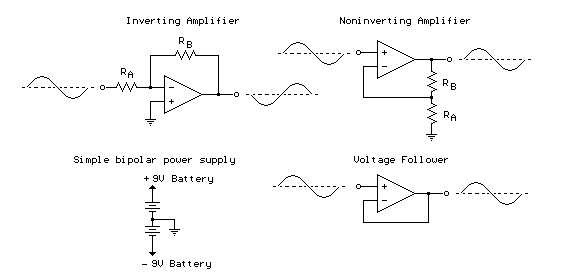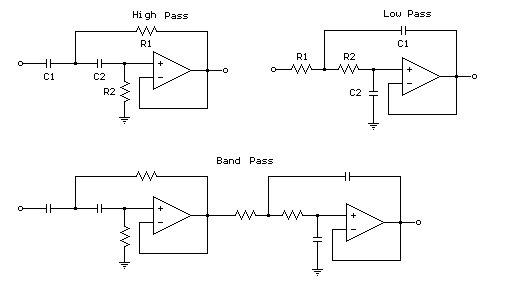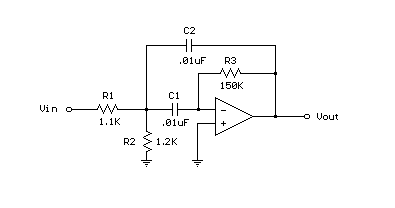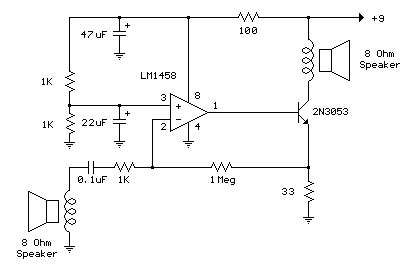### Operational Amplifier (Op-Amp) Basics

The op-amp is basically a differential amplifier having a large voltage gain, very high input impedance and low output impedance. The op-amp has a "inverting" or (-) input and "noninverting" or (+) input and a single output. The op-amp is usually powered by a dual polarity power supply in the range of +/- 5 volts to +/- 15 volts. A simple dual polarity power supply is shown in the figure below which can be assembled with two 9 volt batteries.

Inverting Amplifier:

The op-amp is connected using two resistors RA and RB such that the input signal is applied in series with RA and the output is connected back to the inverting input through RB. The noninverting input is connected to the ground reference or the center tap of the dual polarity power supply. In operation, as the input signal moves positive, the output will move negative and visa versa. The amount of voltage change at the output relative to the input depends on the ratio of the two resistors RA and RB. As the input moves in one direction, the output will move in the opposite direction, so that the voltage at the inverting input remains constant or zero volts in this case. If RA is 1K and RB is 10K and the input is +1 volt then there will be 1 mA of current flowing through RA and the output will have to move to -10 volts to supply the same current through RB and keep the voltage at the inverting input at zero. The voltage gain in this case would be RB/RA or 10K/1K = 10. Note that since the voltage at the inverting input is always zero, the input signal will see a input impedance equal to RA, or 1K in this case. For higher input impedances, both resistor values can be increased.

Noninverting Amplifier:

The noninverting amplifier is connected so that the input signal goes directly to the noninverting input (+) and the input resistor RA is grounded. In this configuration, the input impedance as seen by the signal is much greater since the input will be following the applied signal and not held constant by the feedback current. As the signal moves in either direction, the output will follow in phase to maintain the inverting input at the same voltage as the input (+). The voltage gain is always more than 1 and can be worked out from Vgain = (1+ RB/RA).

Voltage Follower:

The voltage follower, also called a buffer, provides a high input impedance, a low output impedance, and unity gain. As the input voltage changes, the output and inverting input will change by an equal amount.Menu

### 2nd Order Opamp Filters

The figures below illustrate using opamps as active 2nd order filters. Three 2nd order filters are shown, low pass, high pass, and bandpass. Each of these filters will attenuate frequencies outside their passband at a rate of 12dB per octave or 1/4 the voltage amplitude for each octave of frequency increase or decrease outside the passband.

First order low or high pass cutoff frequency (-3dB point) = 1/(2pi*R*C)
2nd order low or high pass cutoff frequency (-3dB point) = 1/2pi(R1*R2*C1*C2)^.5
Example for 2Khz cutoff frequency - R1=R2=7.95K, C1=C2=0.1uFMenu

### Single Op-Amp Bandpass Filter

A bandpass filter passes a range of frequencies while rejecting frequencies outside the upper and lower limits of the passband. The range of frequencies to be passed is called the passband and extends from a point below the center frequency to a point above the center frequency where the output voltage falls about 70% of the output voltage at the center frequency. These two points are not equally spaced above and below the center frequency but will look equally spaced if plotted on a log graph. The percentage change from the lower point to the center will be the same as from the center to the upper, but not the absolute amount. This is similar to a musical keyboard where each key is separated from the next by the same percentage change in frequency, but not the absolute amount.

The filter bandwidth (BW) is the difference between the upper and lower passband frequencies. A formula relating the upper, lower, and center frequencies of the passband is:

Center Frequency = Square Root of (Lower Frequency * Upper Frequency)

The quality factor, or Q of the filter is a measure of the distance between the upper and lower frequency points and is defined as (Center Frequency / BW) so that as the passband gets narrower around the same center frequency, the Q factor becomes higher. The quality factor represents the sharpness of the filter, or rate that the amplitude falls as the input frequency moves away from the center frequency during the first octave. As the frequency gets more than one octave away from center frequency the rollof approaches 6 dB per octave regardless of Q value. Approximate rolloff rates for different Q values for a single octave change from center frequency are:

Q = 1 = 6 dB
Q = 5 = 18 dB
Q = 10 = 24 dB
Q = 50 = 40 dB

For a single op-amp bandpass filter with both capacitors the same value, the Q factor must be greater than the square root of half the gain, so that a gain of 98 would require a Q factor of 7 or more.

The example below shows a 1700 Hz bandpass filter with a Q of 8 and a gain of 65 at center frequency (1700 Hz). Resistor values for the filter can be worked out using the three formulas below. Both capacitor values need to be the same for the formulas to work and are chosen to be 0.01uF which is a common value usable at audio frequencies. This same filter is used in the "Whistle On / Whistle Off" relay toggle circuit.

R1 = Q / (G*C*2*Pi*F) = 8/(65 * .00000001 * 6.28 * 1700) = 1152 or 1.1K
R2 = Q / ((2*Q^2)-G)*C*2*Pi*F) = 8/((128-65) * .00000001 * 6.28 * 1700) = 1189 or 1.2K
R3 = (2*Q) / (C*2*Pi*F) = 16 / (.00000001 * 6.28 * 1700) = 150KMenu

### Low Power Op-Amp - Audio Amp (50 milliwatt)

The example below illustrates using an op-amp as an audio amplifier for a simple intercom. A small 8 ohm speaker is used as a microphone which is coupled to the op-amp input through a 0.1uF capacitor. The speaker is sensitive to low frequencies and the small value capacitor serves to attenuate the lower tones and produce a better overall response. You can experiment with different value capacitors to improve the response for various speakers. The op-amp voltage gain is determined by the ratio of the feedback resistor to the series input resistor which is around one thousand in this case (1 Meg / 1K). The non-inverting input (pin 3) to the op-amp is biased at 50% of the supply voltage (4.5 volts) by a couple 1K resistors connected across the supply. Since both inputs will be equal when the op-amp is operating within it's linear range, the voltage at the non-inverting input (pin 2) and the emitter of the buffer transistor (2N3053) will also be 4.5 volts. The voltage change at the emitter of the transistor will be around +/- 2 volts for a 2 millivolt change at the input (junction of 0.1 cap and 1K resistor) which produces a current change of about 2/33 = 60 mA through the 33 ohm emitter resistor and the speaker output. The peak output speaker power is about I^2 * R or .06 ^2 * 8 = 28 milliwatts. The 100 resistor and 47uF capacitor are used to isolate the op-amp from the power supply and reduce the possibility of oscillation. An additional 22uF cap is used at the non-inverting input to further stabilize operation. These parts may not be needed in such a low power circuit but it's a good idea to decouple the power supply to avoid unwanted feedback. The circuit draws about 1.2 watts from a 9 volt source and is not very efficient but fairly simple to put together. The circuit was tested using a couple 4 inch speakers located a few feet apart (to reduce feedback) and a small pocket transistor radio placed on top of the speaker/microphone as an audio source.Menu

